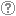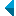# Search the repositoryQuery: search in TitleAuthorAbstractKeywordsFull textYear of publishing ANDORAND NOT search in TitleAuthorAbstractKeywordsFull textYear of publishing ANDORAND NOT search in TitleAuthorAbstractKeywordsFull textYear of publishing ANDORAND NOT search in TitleAuthorAbstractKeywordsFull textYear of publishing Work type: All work types Habilitation (m4) Specialist thesis (m3) High school thesis (m6) Bachelor work * (dip) Master disertations * (mag) Doctorate disertations * (dok) Research Data or Corpuses (data) * old and bologna study programme Language: All languagesSlovenianEnglishGermanCroatianSerbianBosnianBulgarianCzechFinnishFrenchGerman (Austria)HungarianItalianJapaneseLithuanianNorwegianPolishRussianSerbian (cyrillic)SlovakSpanishSwedishTurkishUnknown Search in: RUP    FAMNIT - Faculty of Mathematics, Science and Information Technologies    FHŠ - Faculty of Humanities    FM - Faculty of Management    FTŠ Turistica - Turistica – College of Tourism Portorož    FVZ - Faculty of Health Sciences    IAM - Andrej Marušič Institute    PEF - Faculty of Education    UPR - University of Primorska    ZUP - University of Primorska PressCOBISS    Fakulteta za humanistične študije, Koper    Fakulteta za management Koper in Pedagoška fakulteta Koper    Fakulteta za vede o zdravju, Izola    Knjižnica za tehniko, medicino in naravoslovje, Koper    Turistica, Portorož    Znanstveno-raziskovalno središče Koper Options: Show only hits with full text Reset

 1 - 10 / 19121.Rank-permutable additive mappingsAleksandr Èmilevič Guterman, Anna A. Alieva, Bojan Kuzma, 2006, original scientific articleAbstract: Let ▫$\sigma$▫ be a fixed non-identical permutation on ▫$k$▫ elements. Additive bijections ▫$T$▫ on the matrix algebra ▫$M_n(\mathbb{F})$▫ over a field ▫$\mathbb{F}$▫ of characteristic zero, with the property that ▫$\rm{rk} (A_1...A_k) = \rm{rk} (A_{\sigma(1)}...A_{\sigma(k)})$▫ implies the same condition on the ▫$T$▫ images, are characterized. It is also shown that the surjectivity assumption can be relaxed, if this property is preserved in both directions.Found in: ključnih besedahSummary of found: ...Let ▫$\sigma$▫ be a fixed non-identical permutation on ▫$k$▫ elements. Additive bijections ▫$T$▫ on... Keywords: mathematics, linearna algebra, matrix algebra, rank, permutation, additive preserversPublished: 15.10.2013; Views: 2632; Downloads: 85Full text (0,00 KB) 2.Classification of 2-arc-transitive dihedrantsAleksander Malnič, Shao Fei Du, Dragan Marušič, 2008, original scientific articleAbstract: A complete classification of 2-arc-transitive dihedrants, that is, Cayley graphs of dihedral groups is given, thus completing the study of these graphs initiated by the third author in [D. Marušič, On 2-arc-transitivity of Cayley graphs, J. Combin. Theory Ser. B 87 (2003) 162-196]. The list consists of the following graphs: (i) cycles ▫$C_{2n},\; n \ge 3$▫; (ii) complete graphs ▫$K_{2n}, \; n \ge 3$▫; (iii) complete bipartite graphs ▫$K_{n,n}, \; n \ge 3$▫; (iv) complete bipartite graphs minus a matching ▫$K_{n,n} - nK_2, \; n \ge 3$▫; (v) incidence and nonincidence graphs ▫$B(H_{11})$▫ and ▫$B'(H_{11})$▫ of the Hadamard design on 11 points; (vi) incidence and nonincidence graphs ▫$B(PG(d,q))$▫ and ▫$B'(PG(d,q))$▫, with ▫$d \ge 2$▫ and ▫$q$▫ a prime power, of projective spaces; (vii) and an infinite family of regular ▫${\mathbb{Z}}_d$▫-covers ▫$K_{q+1}^{2d}$▫ of ▫$K_{q+1, q+1} - (q+1)K_2$▫, where ▫$q \ge 3$▫ is an odd prime power and ▫$d$▫ is a divisor of ▫$\frac{q-1}{2}$▫ and ▫$q-1$▫, respectively, depending on whether ▫$q \equiv 1 \pmod{4}$▫ or ▫$q \equiv 3 \pmod{4}$▫ obtained by identifying the vertex set of the base graph with two copies of the projective line ▫$PG(1,q)$▫, where the missing matching consists of all pairs of the form ▫$[i,i']$▫, ▫$i \in PG(1,q)$▫, and the edge ▫$[i,j']$▫ carries trivial voltage if ▫$i=\infty$▫ or ▫$j=\infty$▫, and carries voltage ▫$\bar{h} \in {\mathbb{Z}}_d$▫, the residue class of ▫$h \in {\mathbb{Z}}_d$▫, if and only if ▫$i-j = \theta^h$▫, where ▫$\theta$▫ generates the multiplicative group ▫${\mathbb{F}}_q^\ast$▫ of the Galois field ▫${\mathbb{F}}_q$▫.Found in: ključnih besedahSummary of found: ...permutation group, imprimitive group, dihedral group, Cayley graph,... Keywords: permutation group, imprimitive group, dihedral group, Cayley graph, dihedrant, 2-Arc-transitive graphPublished: 15.10.2013; Views: 2773; Downloads: 85Full text (0,00 KB) 3.Primitive bicirculant association schemes and a generalization of Wielandt's theoremDragan Marušič, István Kovács, Mikhail Muzychuk, 2010, original scientific articleFound in: ključnih besedahSummary of found: ...permutation group, association scheme, primitive association scheme, ... Keywords: permutation group, association scheme, primitive association schemePublished: 15.10.2013; Views: 2611; Downloads: 122Full text (0,00 KB) 4.Decomposition of skew-morphisms of cyclic groupsIstván Kovács, Roman Nedela, 2011, original scientific articleAbstract: A skew-morphism of a group ▫$H$▫ is a permutation ▫$\sigma$▫ of its elements fixing the identity such that for every ▫$x, y \in H$▫ there exists an integer ▫$k$▫ such that ▫$\sigma (xy) = \sigma (x)\sigma k(y)$▫. It follows that group automorphisms are particular skew-morphisms. Skew-morphisms appear naturally in investigations of maps on surfaces with high degree of symmetry, namely, they are closely related to regular Cayley maps and to regular embeddings of the complete bipartite graphs. The aim of this paper is to investigate skew-morphisms of cyclic groups in the context of the associated Schur rings. We prove the following decomposition theorem about skew-morphisms of cyclic groups ▫$\mathbb Z_n$▫: if ▫$n = n_{1}n_{2}$▫ such that ▫$(n_{1}n_{2}) = 1$▫, and ▫$(n_{1}, \varphi (n_{2})) = (\varphi (n_{1}), n_{2}) = 1$▫ (▫$\varphi$▫ denotes Euler's function) then all skew-morphisms ▫$\sigma$▫ of ▫$\mathbb Z_n$▫ are obtained as ▫$\sigma = \sigma_1 \times \sigma_2$▫, where ▫$\sigma_i$▫ are skew-morphisms of ▫$\mathbb Z_{n_i}, \; i = 1, 2$▫. As a consequence we obtain the following result: All skew-morphisms of ▫$\mathbb Z_n$▫ are automorphisms of ▫$\mathbb Z_n$▫ if and only if ▫$n = 4$▫ or ▫$(n, \varphi(n)) = 1$▫.Found in: ključnih besedahSummary of found: ...skew-morphism of a group ▫$H$▫ is a permutation ▫$\sigma$▫ of its elements fixing the identity... Keywords: cyclic group, permutation group, skew-morphism, Schur ringPublished: 15.10.2013; Views: 3099; Downloads: 104Full text (0,00 KB) 5.On cryptographically significant mappings over GF(2 [sup] n)Enes Pašalić, 2008, published scientific conference contributionAbstract: In this paper we investigate the algebraic properties of important cryptographic primitives called substitution boxes (S-boxes). An S-box is a mapping that takes ▫$n$▫ binary inputs whose image is a binary ▫$m$▫-tuple; therefore it is represented as ▫$F:\text{GF}(2)^n \rightarrow \text{GF}(2)^m$▫. One of the most important cryptographic applications is the case ▫$n = m$▫, thus the S-box may be viewed as a function over ▫$\text{GF}(2^n)$▫. We show that certain classes of functions over ▫$\text{GF}(2^n)$▫ do not possess a cryptographic property known as APN (AlmostPerfect Nonlinear) permutations. On the other hand, when ▫$n$▫ is odd, an infinite class of APN permutations may be derived in a recursive manner, that is starting with a specific APN permutation on ▫$\text{GF}(2^k), k$▫ odd, APN permutations are derived over ▫$\text{GF}(2^{k+2i})$▫ for any ▫$i \geq 1$▫. Some theoretical results related to permutation polynomials and algebraic properties of the functions in the ring ▫$\text{GF}(q)[x,y]$▫ are also presented. For sparse polynomials over the field ▫$\text{GF}(2^n)$▫, an efficient algorithm for finding low degree I/O equations is proposed.Found in: ključnih besedahSummary of found: ...cryptographic property known as APN (AlmostPerfect Nonlinear) permutations. On the other hand, when ▫$n$▫ is... Keywords: cryptoanalysis, cryptography, permutation polynomials, power mappings, APN functions, S-box, CCZ-equivalence, algebraic propertiesPublished: 15.10.2013; Views: 2484; Downloads: 68Full text (0,00 KB) 6.Distance-transitive graphs admit semiregular automorphismsKlavdija Kutnar, Primož Šparl, 2010, original scientific articleAbstract: A distance-transitive graph is a graph in which for every two ordered pairs ofvertices ▫$(u,v)$▫ and ▫$(u',v')$▫ such that the distance between ▫$u$▫ and ▫$v$▫ is equal to the distance between ▫$u'$▫ and ▫$v'$▫ there exists an automorphism of the graph mapping ▫$u$▫ to ▫$u'$▫ and ▫$v$▫ to ▫$v'$▫. A semiregular element of a permutation group is anon-identity element having all cycles of equal length in its cycle decomposition. It is shown that every distance-transitive graph admits a semiregular automorphism.Found in: ključnih besedahSummary of found: ...to ▫$v'$▫. A semiregular element of a permutation group is anon-identity element having all cycles... Keywords: distance-transitive graph, vertex-transitive graph, semiregular automorphism, permutation groupPublished: 15.10.2013; Views: 2716; Downloads: 93Full text (0,00 KB) 7.Cubic symmetric graphs via odd automorphisms, 60th Birthday Lecture Series, Department of Mathematics, University of Auckland, New Zealand, 10 September 2015Klavdija Kutnar, 2015, invited lecture at foreign universityFound in: ključnih besedahSummary of found: ...cubic graph, symmetric, automorphism, odd permutation, ... Keywords: cubic graph, symmetric, automorphism, odd permutationPublished: 15.10.2015; Views: 1944; Downloads: 20Full text (0,00 KB) 8.Minimal normal subgroups of transitive permutation groups of square-free degreeAleksander Malnič, Edward Dobson, Dragan Marušič, Lewis A. Nowitz, 2007, original scientific articleAbstract: It is shown that a minimal normal subgroup of a transitive permutation group of square-free degree in its induced action is simple and quasiprimitive, with three exceptions related to ▫$A_5$▫, ▫$A_7$▫, and PSL(2,29). Moreover, it is shown that a minimal normal subgroup of a 2-closed permutation group of square-free degree in its induced action is simple. As an almost immediate consequence, it follows that a 2-closed transitive permutation group of square-free degree contains a semiregular element of prime order, thus giving a partial affirmative answer to the conjecture that all 2-closed transitive permutation groups contain such an element (see [D. Marušic, On vertex symmetric digraphs,Discrete Math. 36 (1981) 69-81; P.J. Cameron (Ed.), Problems from the fifteenth British combinatorial conference, Discrete Math. 167/168 (1997) 605-615]).Found in: ključnih besedahSummary of found: Zadetek v naslovuKeywords: mathematics, graph theory, transitive permutation group, 2-closed group, square-free degree, semiregular automorphism, vertex-transitive graphPublished: 03.04.2017; Views: 1827; Downloads: 85Full text (0,00 KB) 9.Semiregular automorphisms of vertex-transitive graphs of certain valenciesAleksander Malnič, Edward Dobson, Dragan Marušič, Lewis A. Nowitz, 2007, original scientific articleAbstract: It is shown that a vertex-transitive graph of valency ▫$p+1$▫, ▫$p$▫ a prime, admitting a transitive action of a ▫$\{2,p\}$▫-group, has a non-identity semiregular automorphism. As a consequence, it is proved that a quartic vertex-transitive graph has a non-identity semiregular automorphism, thus giving a partial affirmative answer to the conjecture that all vertex-transitive graphs have such an automorphism and, more generally, that all 2-closed transitive permutation groups contain such an element (see [D. Marušic, On vertex symmetric digraphs, Discrete Math. 36 (1981) 69-81; P.J. Cameron (Ed.), Problems from the Fifteenth British Combinatorial Conference, Discrete Math. 167/168 (1997) 605-615]).Found in: ključnih besedahSummary of found: ...and, more generally, that all 2-closed transitive permutation groups contain such an element (see [D.... Keywords: mathematics, graph theory, transitive permutation group, 2-closed group, semiregular automorphism, vertex-transitive graphPublished: 03.04.2017; Views: 1882; Downloads: 78Full text (0,00 KB) 10.Monomial isomorphisms of cyclic codesEdward Dobson, 2015, original scientific articleFound in: ključnih besedahSummary of found: ...Cyclic code, Monomial, Permutation, Isomorphism, Equivalent, ... Keywords: Cyclic code, Monomial, Permutation, Isomorphism, EquivalentPublished: 03.04.2017; Views: 1897; Downloads: 129Full text (0,00 KB)
Search done in 0 sec.